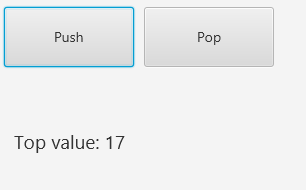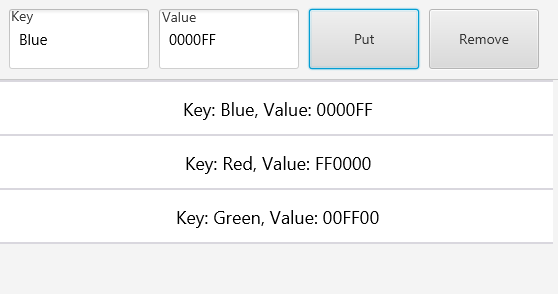# 👩‍🏫 👨‍🏫 Examples for teachers - Algorithms

Status
Not open for further replies.

#### Erel

Staff member
We want to help teachers teach programming and/or computer science with B4X.
In this thread I will try to build a collection of classic algorithms that teachers can use as part of their lessons.

The first few examples are well explained in Jeff Erickson's Algorithms book: http://jeffe.cs.illinois.edu/teaching/algorithms/
The book material is quite advanced however the first chapters are not too complicated.

To keep this thread "clean", it is closed for discussions. You are welcome to post here: https://www.b4x.com/android/forum/threads/--examples-for-teachers-discuss-here.115896/

Algorithms and data structures index:

The examples in this thread are shared in the public domain. You can do whatever you like with them.

Last edited:
•••mohsyn, XToolsGroup, muhammadali213123 and 31 others

#### Erel

Staff member
Tower of Hanoi - example that shows the magic and power of recursion.
The puzzle is explained here: https://www.b4x.com/guides/Algorithms-JeffE_Recursion/?page=42

The puzzle is solved in about three lines:
B4X:
``````Sub Hanoi (n As Int, SrcPeg As Peg, DestPeg As Peg, TempPeg As Peg) As ResumableSub
If n > 0 Then
Wait For (Hanoi(n - 1, SrcPeg, TempPeg, DestPeg)) Complete (unused As Boolean)
Wait For (MoveDisk(SrcPeg, DestPeg)) Complete (unused As Boolean)
Wait For (Hanoi(n - 1, TempPeg, DestPeg, SrcPeg)) Complete (unused As Boolean)
End If
Return True
End Sub``````

The reason that I'm using Wait For to make the calls is that each step is animated. This way the code will wait for the called sub to complete (this is always the case when calling non-resumable subs).B4J project is attached.

#### Attachments

• TowerOfHanoi.zip
2.9 KB · Views: 381
Last edited:

#### Erel

Staff member
Merge Sort - recursive sorting based on merging sorted sub-arrays.
Explained here: https://www.b4x.com/guides/Algorithms-JeffE_Recursion/?page=44

The differences in the code compared to the pseudo code are:
- The "StartIndex" position is passed instead of creating sub-arrays.
- The arrays indices start from 0.
- The array holds a custom type with the numeric value and the label.
- The sub calls are done with Wait For to let the animations complete.B4J project is attached.

#### Attachments

• MergeSort.zip
2.7 KB · Views: 313
Last edited:

#### Erel

Staff member
Quick sort - Sorting based on partitioning. It works in the opposite way compared to merge sort.
The pivot is selected randomly. It works best when the pivot is close to the median number.

Explanation: https://www.b4x.com/guides/Algorithms-JeffE_Recursion/?page=47B4J project is attached.

#### Attachments

• QuickSort.zip
2.7 KB · Views: 291
Last edited:

#### Erel

Staff member
SplitMultiply - Fast multiplication algorithm for multiplying two n digits numbers.
The numbers are stored as strings.
We only use the addition operator and multiplication of one digit numbers.
Explanation: https://www.b4x.com/guides/Algorithms-JeffE_Recursion/?page=58

(this is of course millions of times slower than using the built in hardware based math features)

B4X code:
B4X:
``````'Non-UI application (console / server application)
Sub AppStart (Args() As String)
Log(SplitMultiply("1234", "0002", 4))
Log(SplitMultiply("80001", "12341", 5))
End Sub

Sub SplitMultiply (x As String, y As String, n As Int) As String
If n = 1 Then
Return GetDigitFromString(x, 0) * GetDigitFromString(y, 0) 'one digit * one digit
Else
Dim m As Int = Ceil(n / 2)
Dim a As String = DivideBy10M(x, m)
Dim b As String = Modulo10M(x, m)
Dim c As String = DivideBy10M(y, m)
Dim d As String = Modulo10M(y, m)
Dim e As String = SplitMultiply(a, c, m)
Dim f As String = SplitMultiply(b, d, m)
Dim g As String = SplitMultiply(b, c, m)
Dim h As String = SplitMultiply(a, d, m)
End If
'the strings will be parsed here and later converted back to a string.
'we use NumberFormat2 to control the formatting.
Return NumberFormat2(ShiftByM(e, 2 * m) + ShiftByM(g + h, m) + f, 0, 0, 0, False)
End Sub

Sub GetDigitFromString(s As String, index As Int) As Int
Return "" & s.CharAt(index)
End Sub

Sub DivideBy10M (s As String, m As Int) As String
If s.Length <= m Then Return "0"
Return s.SubString2(0, s.Length - m)
End Sub

Sub Modulo10M (s As String, m As Int) As String
Return s.SubString(s.Length - m)
End Sub

Sub ShiftByM(s As String, m As Int) As String
For i = 0 To m - 1
s = s & "0"
Next
Return s
End Sub``````

#### Erel

Staff member
Insertion Sort - Simple iterative algorithm. On each step we find the position of the next element in the previously sorted items.

Explanation: https://en.wikipedia.org/wiki/Insertion_sort#### Attachments

• InsertionSort.zip
2.6 KB · Views: 299

Staff member

#### Attachments

• N Queens.zip
2.8 KB · Views: 297
••XToolsGroup, Sureshv2, maXim and 6 others

#### Erel

Staff member
ConstructSubset - Recursively finds a subset of values that add up to the target value.

Explanation: https://www.b4x.com/guides/Algorithms-JeffE_Backtracking/?page=6

The algorithm was adjusted to avoid making copies of the list.
Example:
X: {8, 6, 7, 5, 3, 10, 9}
Target: 33
Output: 6 + 5 + 3 + 10 + 9 = 33

B4X:
``````'Non-UI application (console / server application)
Sub AppStart (Args() As String)
Dim x As List
x.Initialize
x.AddAll(Array As Int(8, 6, 7, 5, 3, 10, 9)) 'we cannot use x = Array(..) as it will create a read-only list.
Dim Target As Int = 33
If ConstructSubset(x, Target) Then
Dim sb As StringBuilder
sb.Initialize
For Each v As Int In x
If sb.Length > 0 Then sb.Append(" + ")
sb.Append(v)
Next
sb.Append(" = ").Append(Target)
Log(sb.ToString)
Else
Log("No solution")
End If
End Sub

'Finds a single subset that add up to Target.
'Modifies the input list.
Sub ConstructSubset (X As List, Target As Int) As Boolean
If Target = 0 Then
'remove all remaining values
X.Clear
Return True
End If
If Target < 0 Or X.Size = 0 Then
Return False
End If
Dim CurrentValue As Int = X.Get(0)
X.RemoveAt(0)
'without current value
Dim res As Boolean = ConstructSubset(X, Target)
If res Then Return True
'with current value
res = ConstructSubset(X, Target - CurrentValue)
'put the value back in the list
X.InsertAt(0, CurrentValue)
Return res
End Sub``````

Last edited:

#### Erel

Staff member
Singly linked list implemented in a class.#### Attachments

3 KB · Views: 284

#### Erel

Staff member
Stack data structure (LIFO = Last In First Out). I'm using the built-in List collection to store the elements for simplicity. The same code would have worked with the previously implemented LinkedList.

Explanation: https://en.wikipedia.org/wiki/Stack_(abstract_data_type)#### Attachments

• Stack.zip
2.4 KB · Views: 247

#### Erel

Staff member
Hash Table / Map / Dictionary / Associated Array data structure. Implementation of the beloved Map collection. It is a bit more complicated than the two previous data collections.

Explanation: https://en.wikipedia.org/wiki/Hash_tableThe buckets are made of list of items. The bucket is chosen based on the string hash value.
Quiz: what will happen if we change CalcHash to always return 5 ? Will it work properly?

#### Attachments

• HashTable.zip
3.7 KB · Views: 289

#### Erel

Staff member
Binary Search Tree (BST) data structure - a binary tree where larger values are on the right side and smaller values are on the left side. This is an efficient structure that allows getting the values in sorted order.
It is also a good example of working with trees where almost all tasks are done recursively.
Explanation: https://en.wikipedia.org/wiki/Binary_search_tree#### Attachments

• BinarySearchTree.zip
4.1 KB · Views: 289
Status
Not open for further replies.

Replies
5
Views
2K
Replies
5
Views
1K
Replies
41
Views
11K
Replies
20
Views
3K
Replies
5
Views
2K